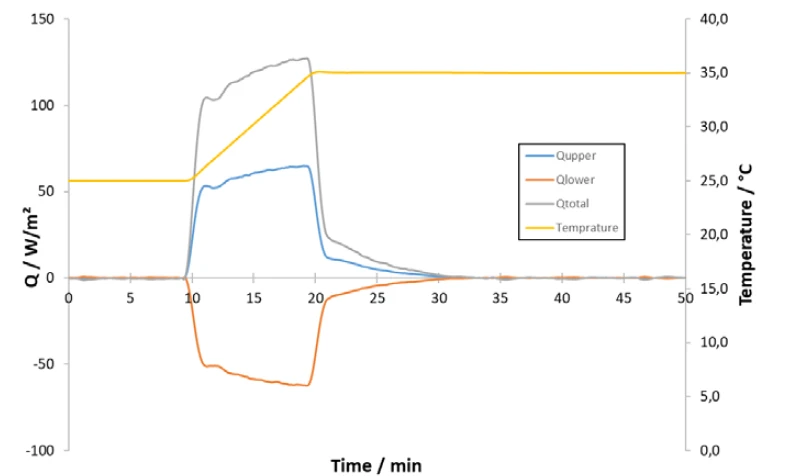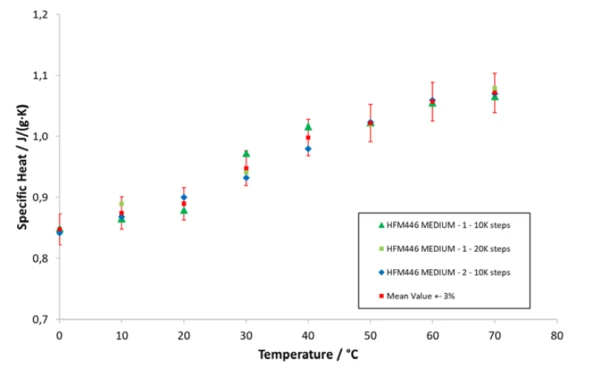# Easy Determination of the Specific Heat Capacity of Glass Wool by Means of HFM Measurements

#### Measurement Conditions

For measurement of the Specific Heat Capacity (cp)Heat capacity is a material-specific physical quantity, determined by the amount of heat supplied to specimen, divided by the resulting temperature increase. The specific heat capacity is related to a unit mass of the specimen.specific heat capacity, Specific Heat Capacity (cp)Heat capacity is a material-specific physical quantity, determined by the amount of heat supplied to specimen, divided by the resulting temperature increase. The specific heat capacity is related to a unit mass of the specimen.cp, with the NETZSCH HFM 446 Lambda, both plates are held at exactly the same temperature. When there is no more heat flux between the two plates, a temperature step is initialized. Heat-flux transducers measure the resulting heat flux into the sample; the signal is then integrated and evaluated. By performing a so-called empty stack measurement (system without sample) prior to the sample measurement, the specific heat of the system is taken into consideration. The NETZSCH HFM 446 LambdaSmall and Medium can measure the Specific Heat Capacity (cp)Heat capacity is a material-specific physical quantity, determined by the amount of heat supplied to specimen, divided by the resulting temperature increase. The specific heat capacity is related to a unit mass of the specimen.specific heat capacity of solid polymers such as polyamide or PVC, and of insulation materials such as glass wool.

#### Specific Heat Capacity of a Glass Fiber Insulation

Not only the thermal conductvity but also the Specific Heat Capacity (cp)Heat capacity is a material-specific physical quantity, determined by the amount of heat supplied to specimen, divided by the resulting temperature increase. The specific heat capacity is related to a unit mass of the specimen.specific heat capacity of insulation materials is an important material property in the building sector. The SI unit for the Specific Heat Capacity (cp)Heat capacity is a material-specific physical quantity, determined by the amount of heat supplied to specimen, divided by the resulting temperature increase. The specific heat capacity is related to a unit mass of the specimen.specific heat capacity is J/(g∙K). It gives information about the amount of energy necessary in Joules to heat up 1 gram of the material by 1 degree Kelvin. Insulation materials with high specific heat capacities can dampen temperature extremes in the outer environment and contribute to a steady indoor climate. Still one of the most important insulation materials is glass wool. In the following example, glass wool was investigated with two different NETZSCH HFM 446 LambdaMedium devices (for reproducibility) within a temperature range of 0°C to 70°C using different temperature steps (10 K and 20 K). The size of the sample was approx. 30 cm x 30 cm x 2.5 cm with a mass of approx. 300 g.

Figure 1 shows the measurement signals recorded during the measurement of the glass wool sample of the temperature and heat flux of one temperature step from 25°C to 35°C versus timewere. The resulting combined heat flux (Qtotal) of the upper and lower plates (Qupper and Qlower) represents the total heat consumption necessary to heat up the sample (including the plates). Based on the integral and the empty stack measurement previously carried out, the Specific Heat Capacity (cp)Heat capacity is a material-specific physical quantity, determined by the amount of heat supplied to specimen, divided by the resulting temperature increase. The specific heat capacity is related to a unit mass of the specimen.specific heat capacity at a mean temperature of 30°C can be determined.

Figure 2 shows the Specific Heat Capacity (cp)Heat capacity is a material-specific physical quantity, determined by the amount of heat supplied to specimen, divided by the resulting temperature increase. The specific heat capacity is related to a unit mass of the specimen.specific heat capacity of a glass fiber insulation between 0°C and 70°C. The specific heat rises with increasing temperature. The results of all measurements vary within ± 3% around the mean value and are withing the expected range for glass fiber insulations (<1 J/(g·K) at room temperature).1) Heat flux and temperature of a temperature step during the Specific Heat Capacity (cp)Heat capacity is a material-specific physical quantity, determined by the amount of heat supplied to specimen, divided by the resulting temperature increase. The specific heat capacity is related to a unit mass of the specimen.cp measurement by means of the NETZSCH-HFM 446 Lamda Medium2) Specific Heat Capacity (cp)Heat capacity is a material-specific physical quantity, determined by the amount of heat supplied to specimen, divided by the resulting temperature increase. The specific heat capacity is related to a unit mass of the specimen.Specific heat capacity of a glass fiber insulation between 0° C and 70°C

#### Summary

These measurements clearly demonstrate that the NETZSCH HFM 446 Lambda is capable of determining the Specific Heat Capacity (cp)Heat capacity is a material-specific physical quantity, determined by the amount of heat supplied to specimen, divided by the resulting temperature increase. The specific heat capacity is related to a unit mass of the specimen.specific heat capacity of large-volume and inhomogeneous materials typcially for applications in the building and insulation industries.